2

135

4四处流浪
328/500

13986

56主题 328积分UID
5

1

1113

0

2013-5-24

65 小时

2019-10-172019-9-27 11:29:23 显示全部楼层 阅读模式

马上注册，结交更多好友，享用更多功能，让你轻松玩转社区。

x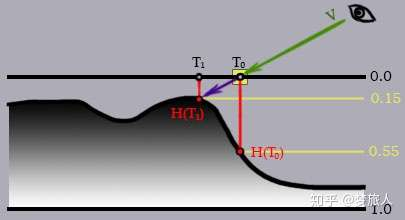（1）从高度图读取纹理坐标T0位置的高度H(T0)
（2）根据H(T0)和摄像机向量V，在初始的纹理坐标基础上进行偏移。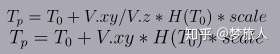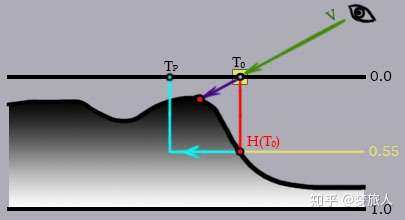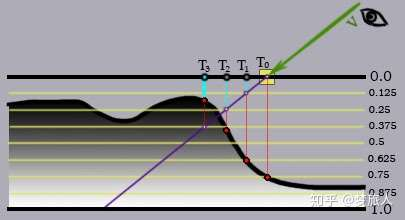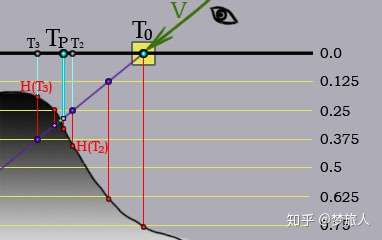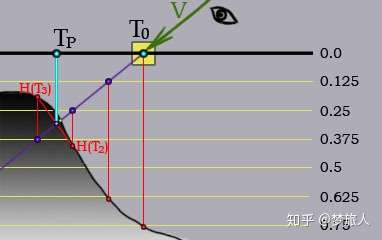VS方法
[C#] 纯文本查看 复制代码
v2f vert (appdata_full v)[/size]
[size=4]{[/size]
[size=4]    v2f o;[/size]
[size=4]    o.pos = UnityObjectToClipPos(v.vertex);[/size]
[size=4]    o.uv = TRANSFORM_TEX(v.texcoord,_MainTex) + frac(_Time.y*_HeightTileSpeed.zw);[/size]
[size=4]    o.uv2 = v.texcoord * _HeightTileSpeed.xy;[/size]
[size=4]    o.posWorld = mul(unity_ObjectToWorld, v.vertex);[/size]
[size=4]    o.normalDir = UnityObjectToWorldNormal(v.normal);[/size]
[size=4]    TANGENT_SPACE_ROTATION;[/size]
[size=4]    o.viewDir = mul(rotation, ObjSpaceViewDir(v.vertex));[/size]
[size=4]    o.color = v.color;[/size]
[size=4]#if USING_FOG[/size]
[size=4]    HeightFog(o.posWorld.xyz,o.fog);[/size]
[size=4]#endif[/size]
[size=4]    UNITY_TRANSFER_FOG(o,o.pos);[/size]
[size=4]    return o;[/size]
[size=4]}[/size]

[size=4]

viewDir是切线空间下的视线向量，透明度写在顶点色里
FS方法里初始化
[C#] 纯文本查看 复制代码
float3 viewRay = normalize(-i.viewDir);
viewRay.z = abs(viewRay.z)+0.42;
viewRay.xy *= _Height;

float3 shadeP = float3(i.uv,0);
float3 shadeP2 = float3(i.uv2,0);

0.42的一个hack的值，是为了防止摄像机向量V和法向量N夹角过大。本身精度足够是不需要这个值的，但是为了减少迭代次数必须加上这个值。
_Height控制云的凹凸程度。

RPM版

[C#] 纯文本查看 复制代码
const int linearStep = 2;
const int binaryStep = 5;
float4 T = tex2D(_MainTex, shadeP2.xy);
float h2 = T.a * _HeightAmount;
// linear search
float3 lioffset = viewRay / (viewRay.z * (linearStep+1));
for(int k=0; k<linearStep; k++)
{
float d = 1.0 - tex2Dlod(_MainTex, float4(shadeP.xy,0,0)).a * h2;
}
// binary search
float3 biOffset = lioffset;
for(int j=0; j<binaryStep; j++)
{
biOffset = biOffset * 0.5;
float d = 1.0 - tex2Dlod(_MainTex, float4(shadeP.xy,0,0)).a * h2;
shadeP += biOffset * sign(d - shadeP.z);
}

POM版
[C#] 纯文本查看 复制代码
float linearStep = 7;[/size]
[size=4]float4 T = tex2D(_MainTex, shadeP2.xy);[/size]
[size=4]float h2 = T.a * _HeightAmount;[/size]
[size=4]float3 lioffset = viewRay / (viewRay.z * linearStep);[/size]
[size=4]float d = 1.0 - tex2Dlod(_MainTex, float4(shadeP.xy,0,0)).a * h2;[/size]
[size=4]float3 prev_d = d;[/size]
[size=4]{[/size]
[size=4]    shadeP += lioffset;[/size]
[size=4]    prev_d = d;[/size]
[size=4]    d = 1.0 - tex2Dlod(_MainTex, float4(shadeP.xy,0,0)).a * h2;[/size]
[size=4]}[/size]
[size=4]float d1 = d - shadeP.z;[/size]
[size=4]float d2 = prev_d - prev_shadeP.z;[/size]
[size=4]float w = d1 / (d1 - d2);[/size]
[size=4]

[C#] 纯文本查看 复制代码
half4 c = tex2D(_MainTex,shadeP.xy) * T * _Color;[/size]
[size=4]half Alpha = i.color.r;[/size]
[size=4]float3 normal = normalize(i.normalDir);[/size]
[size=4]half3 lightDir = UnityWorldSpaceLightDir(i.posWorld);[/size]
[size=4]float NdotL = max(0,dot(normal,lightDir));[/size]
[size=4] [/size]
[size=4]#if USING_FOG[/size]
[size=4]    fixed3 viewDir = normalize(UnityWorldSpaceViewDir(i.posWorld));[/size]
[size=4]    float sunFog = saturate( dot(-viewDir,lightDir));[/size]
[size=4]    half3 sunFogColor  = lerp(_HeightFogColor,_sunFogColor,pow(sunFog,2));[/size]
[size=4]    fixed3 finalColor = c.rgb * (NdotL * lightColor + unity_AmbientEquator.rgb * sunFogColor * _LightIntensity);[/size]
[size=4]    unity_FogColor.rgb = lerp(sunFogColor, unity_FogColor.rgb, i.fog.y*i.fog.y);[/size]
[size=4]    finalColor.rgb = lerp(finalColor.rgb,unity_FogColor.rgb, i.fog.x);[/size]
[size=4]#else[/size]
[size=4]    fixed3 finalColor = c.rgb*(NdotL*lightColor + unity_AmbientEquator.rgb);[/size]
[size=4]#endif[/size]
[size=4]UNITY_APPLY_FOG(i.fogCoord, finalColor);[/size]
[size=4] [/size]
[size=4]return ColorOutput(fixed4(finalColor.rgb,Alpha));[/size]
[size=4]

2初来乍到
116/150

28932

0主题 116积分UID
55623

0

14

0

2014-11-15

42 小时

2019-10-162019-10-15 15:32:47 显示全部楼层
 牛皮反复发生范德萨发大水发射点发射点

0主题 34积分UID
281120

0

9

0

2018-5-14

18 小时

2019-10-226 天前 显示全部楼层
 厉害厉害

 本版积分规则 回帖后跳转到最后一页